# RD Sharma Solutions for Class 10 Maths Chapter 1 Real Numbers Exercise 1.4

Applications of the Fundamental Theorem of Arithmetic are finding the LCM and HCF of positive integers. So, this exercise deals with problems in finding the LCM and HCF by prime factorisation method. Also, the relationship between LCM and HCF is understood in the RD Sharma Solutions Class 10 Exercise 1.4. For quick access to complete solutions of this exercise, the RD Sharma Solutions for Class 10 Maths Chapter 1 Real Numbers Exercise 1.4 PDF is available below.

## RD Sharma Solutions for Class 10 Maths Chapter 1 Real Numbers Exercise 1.4 Download PDF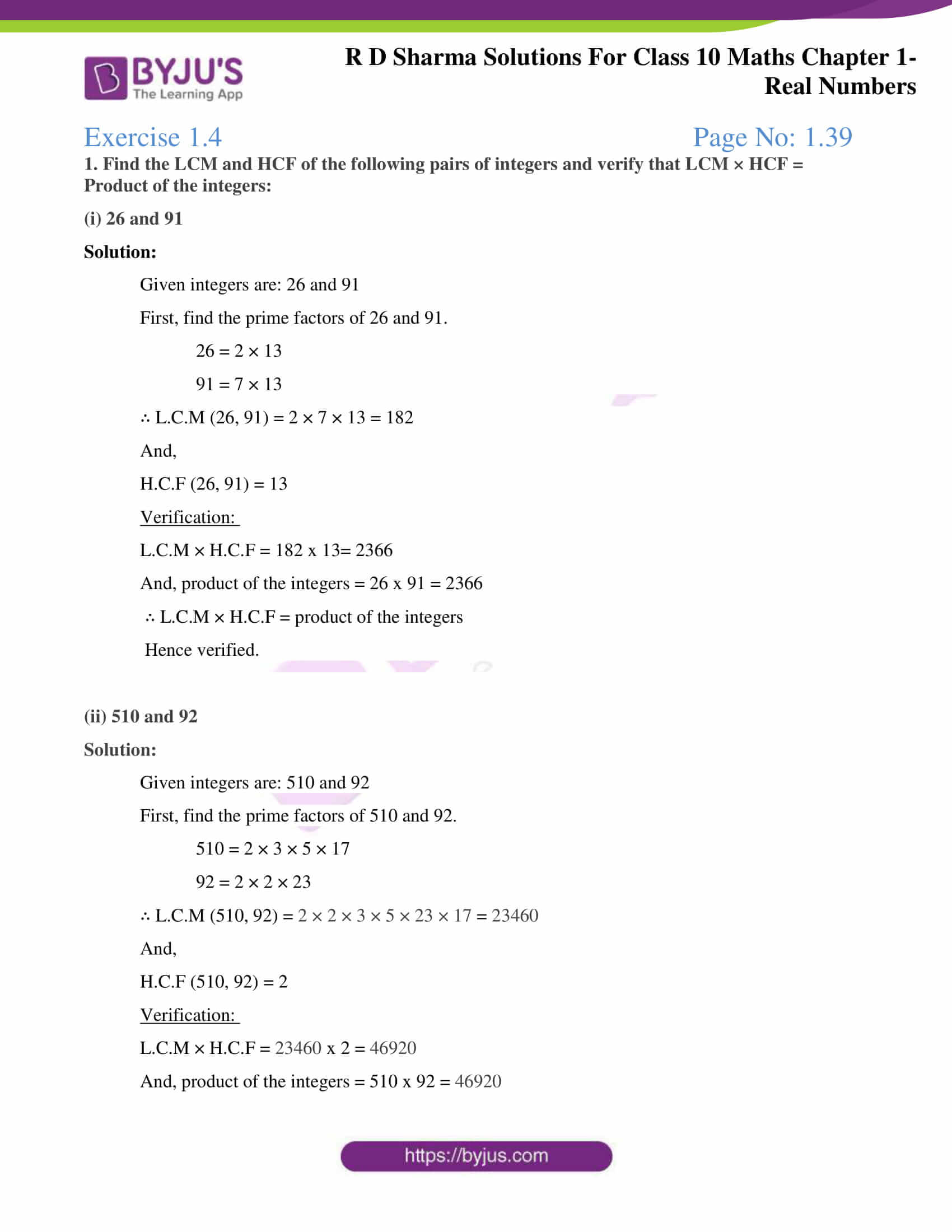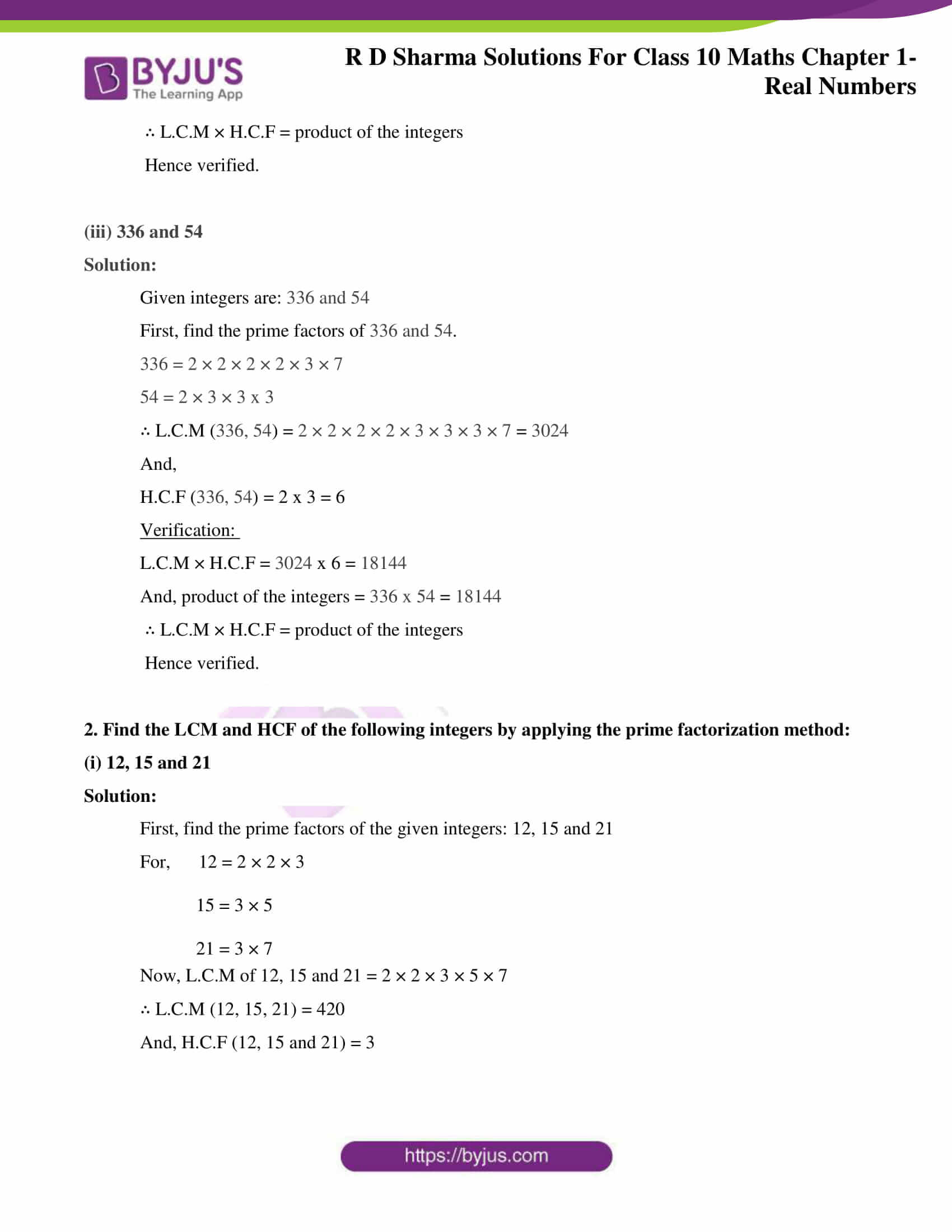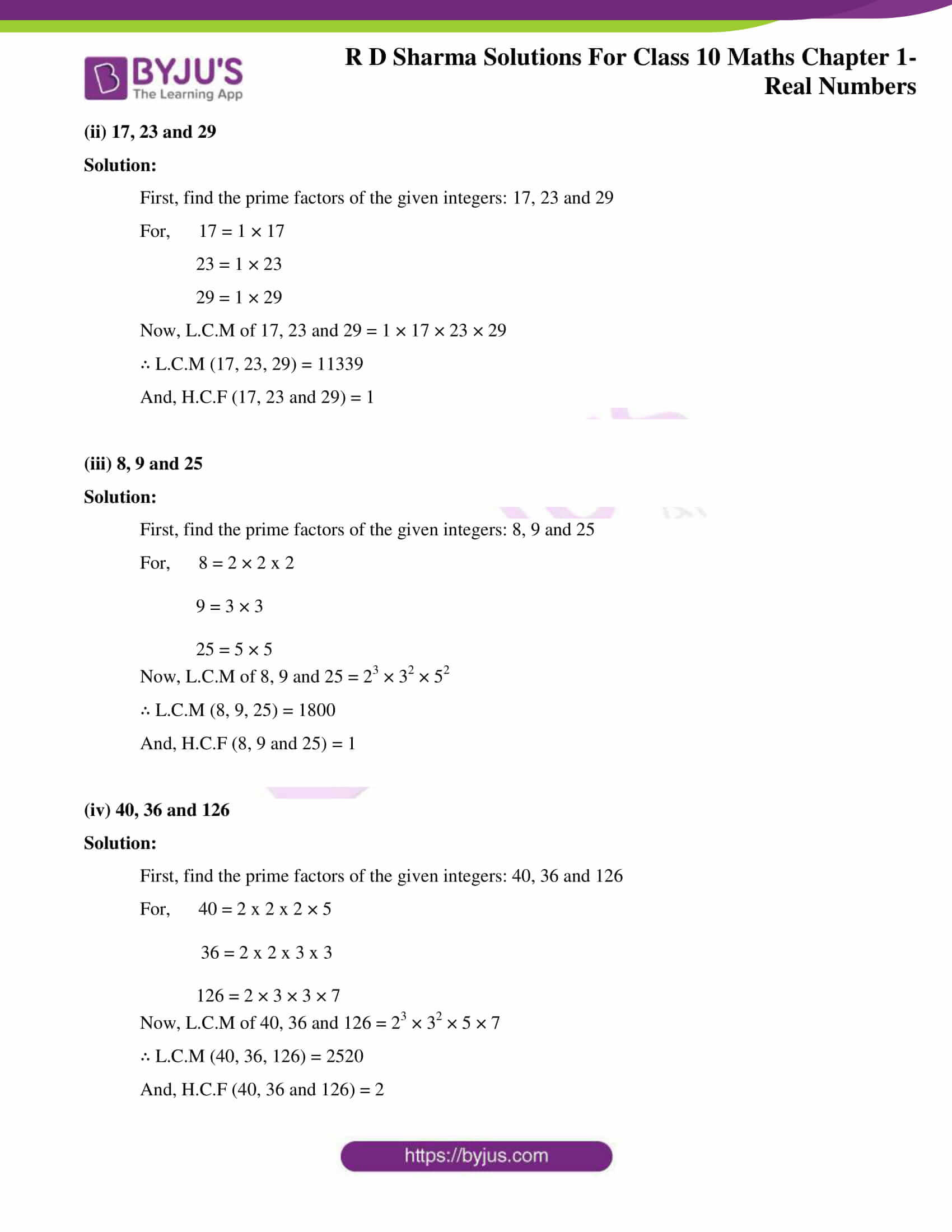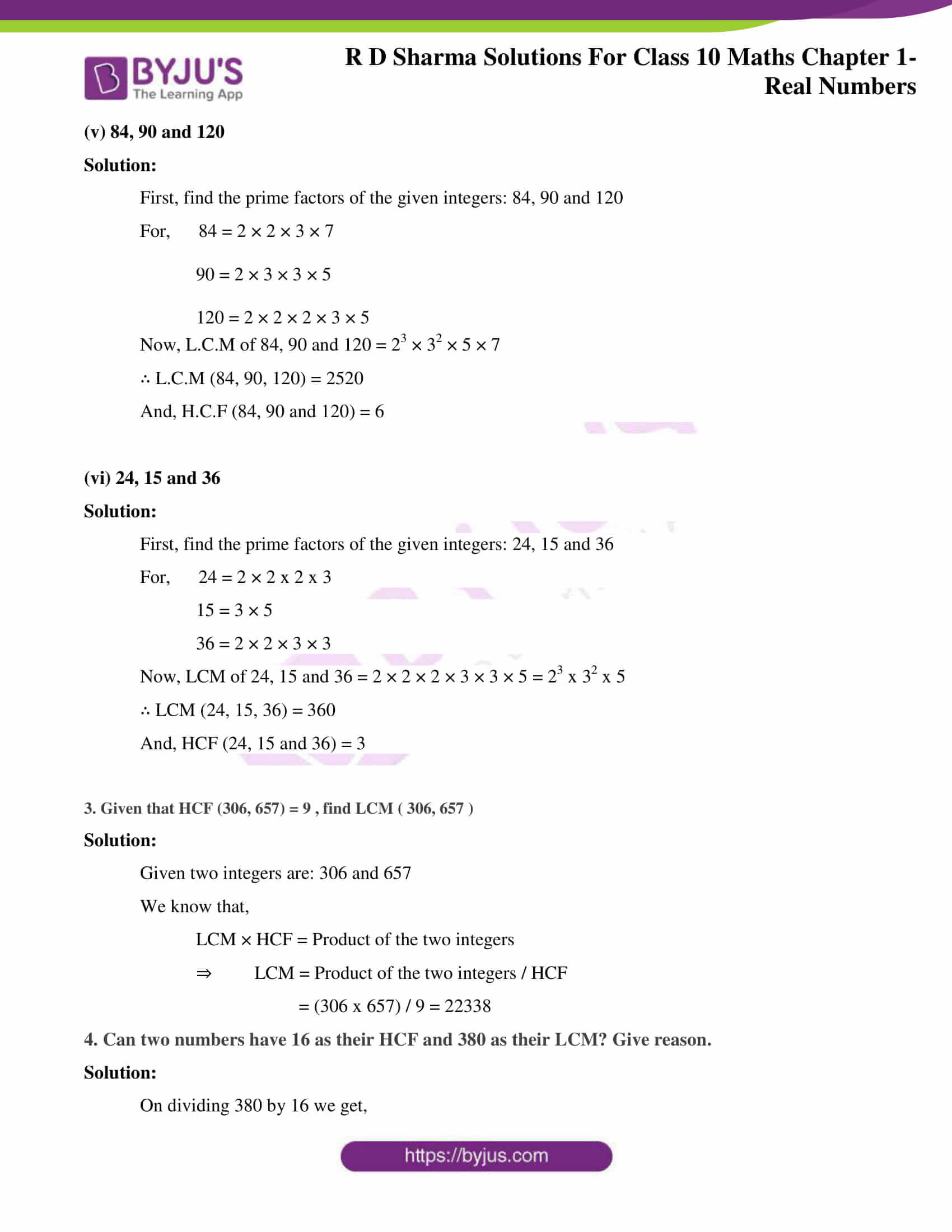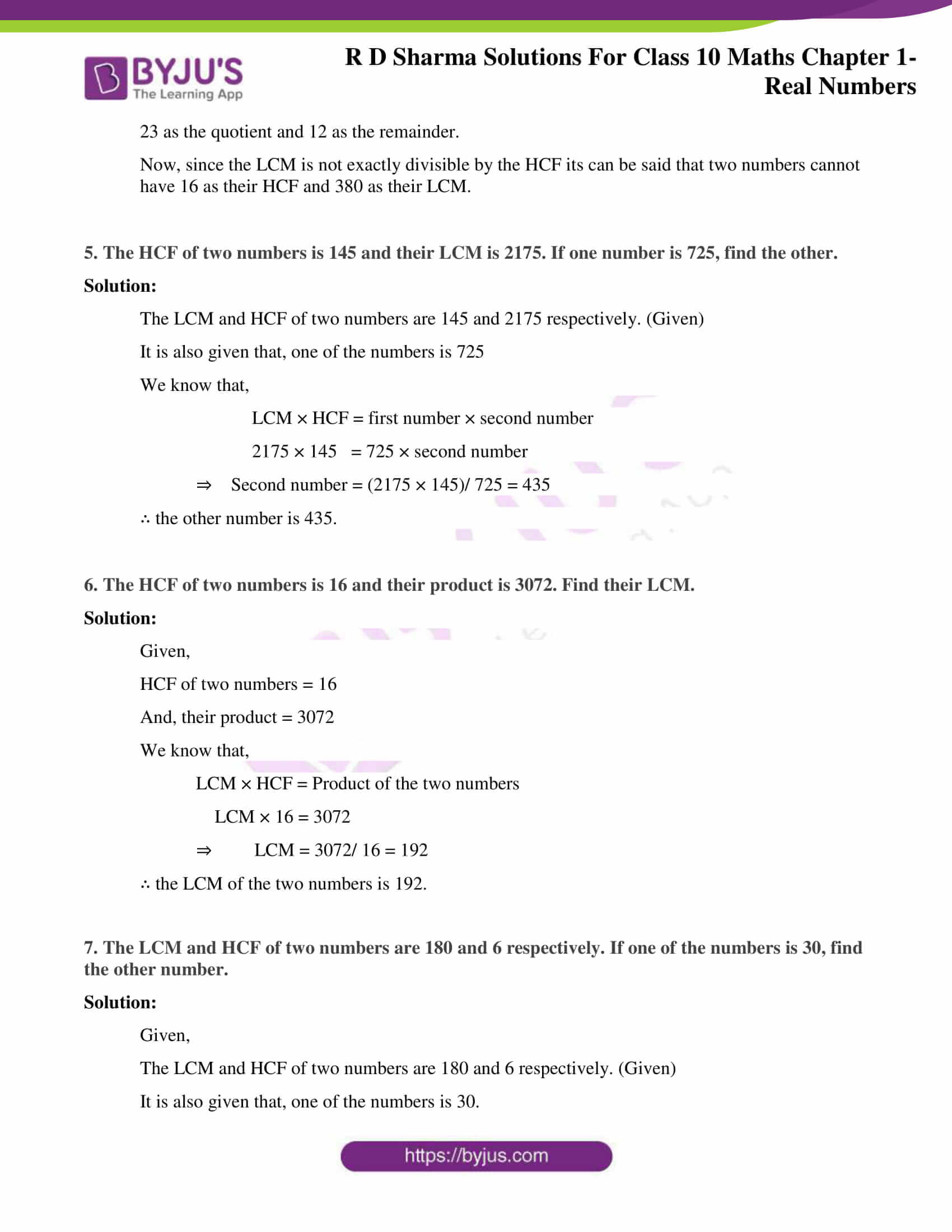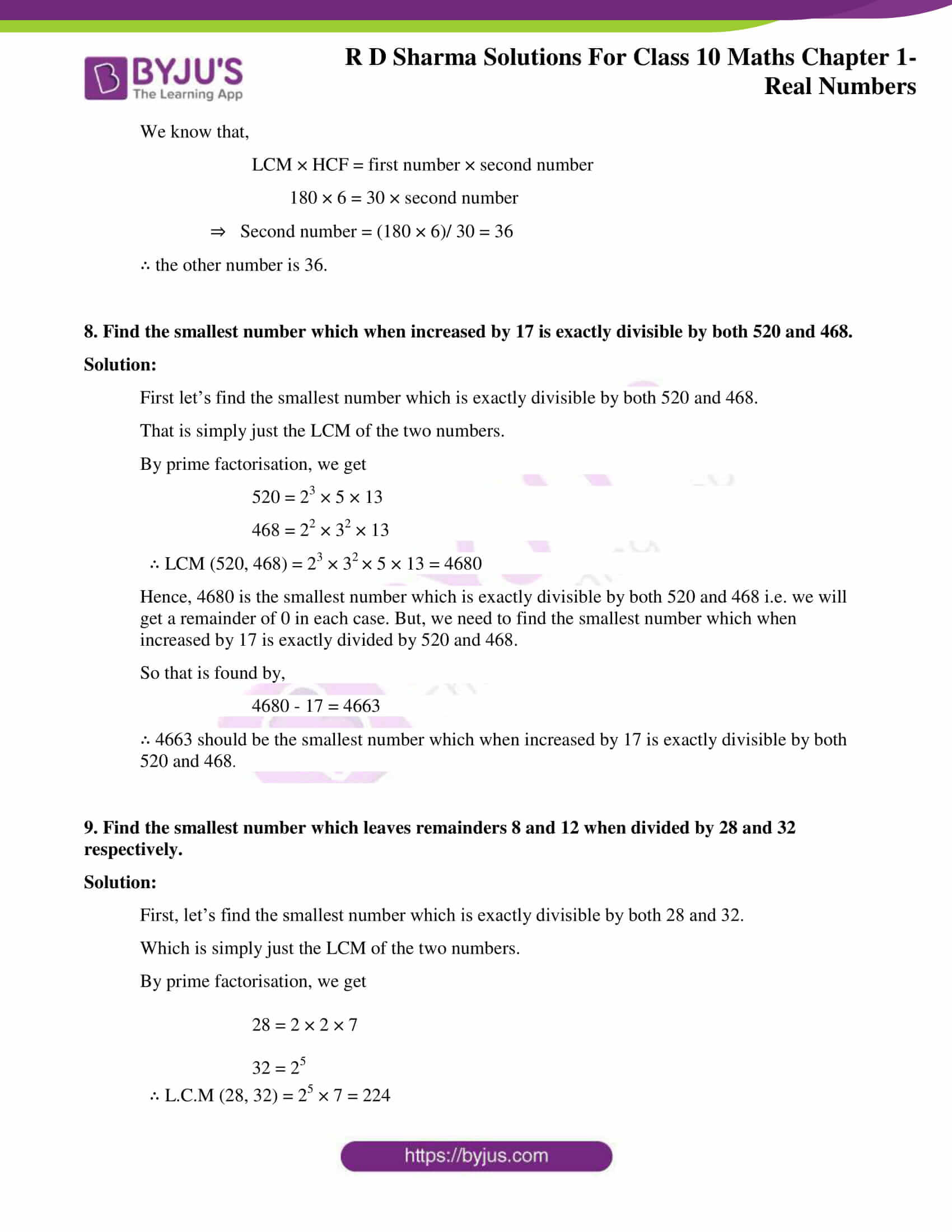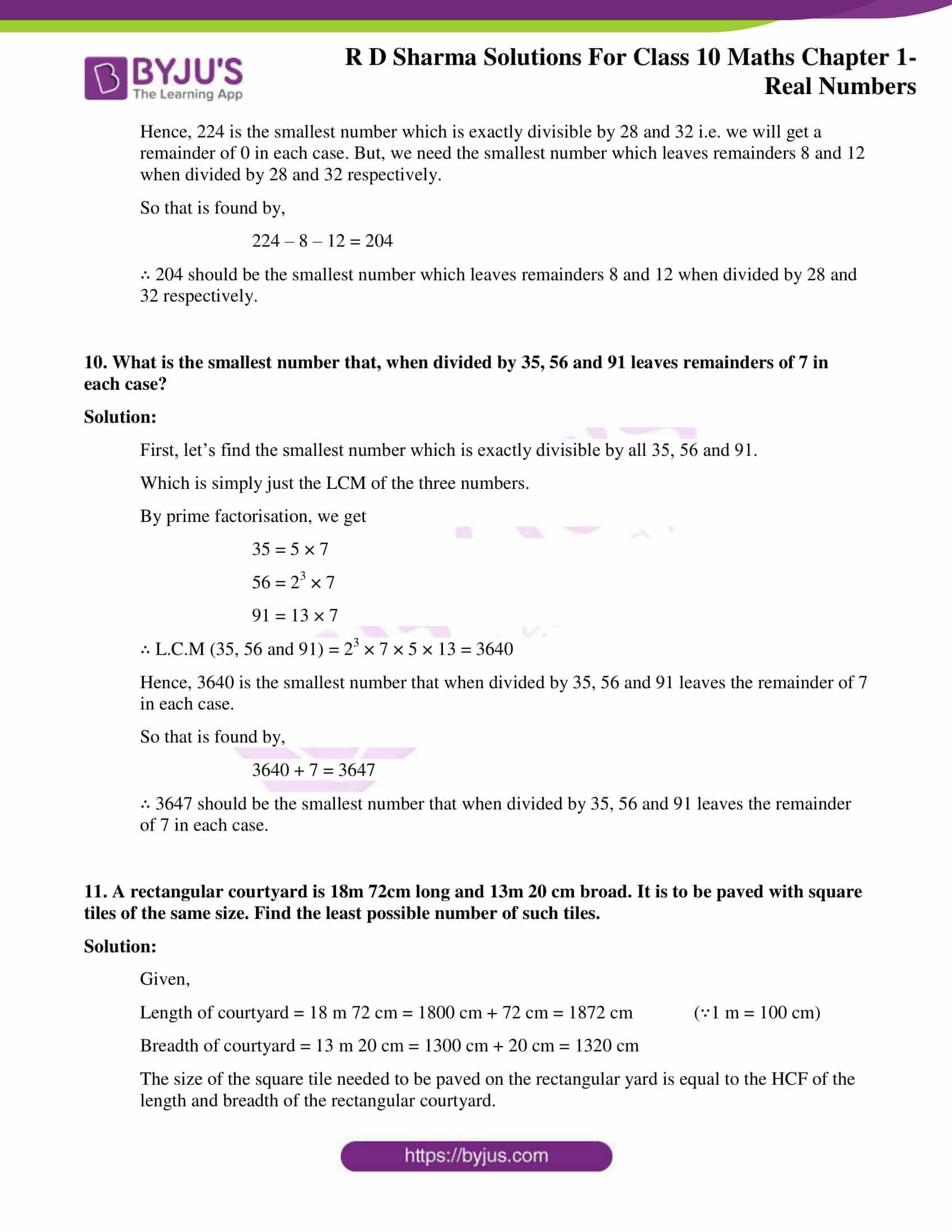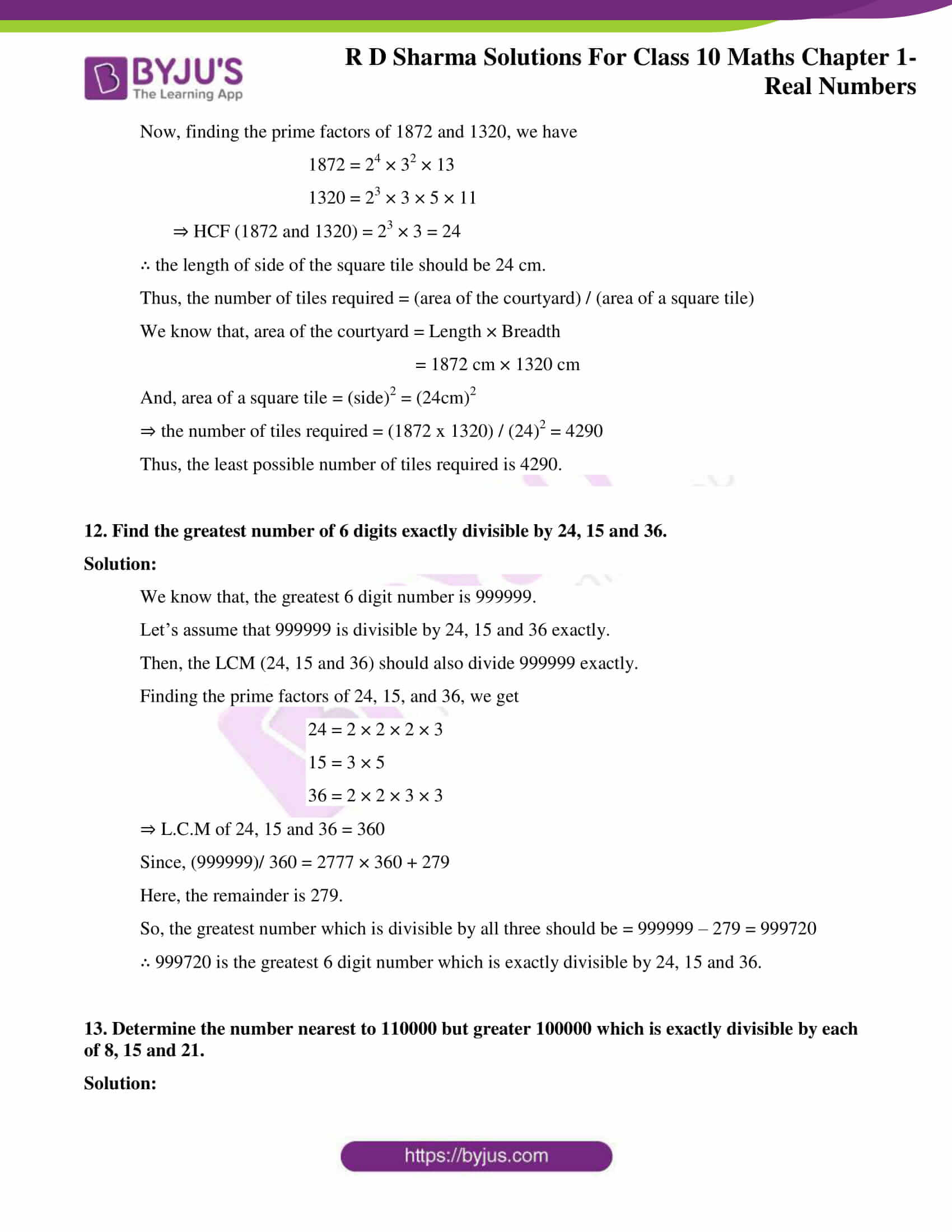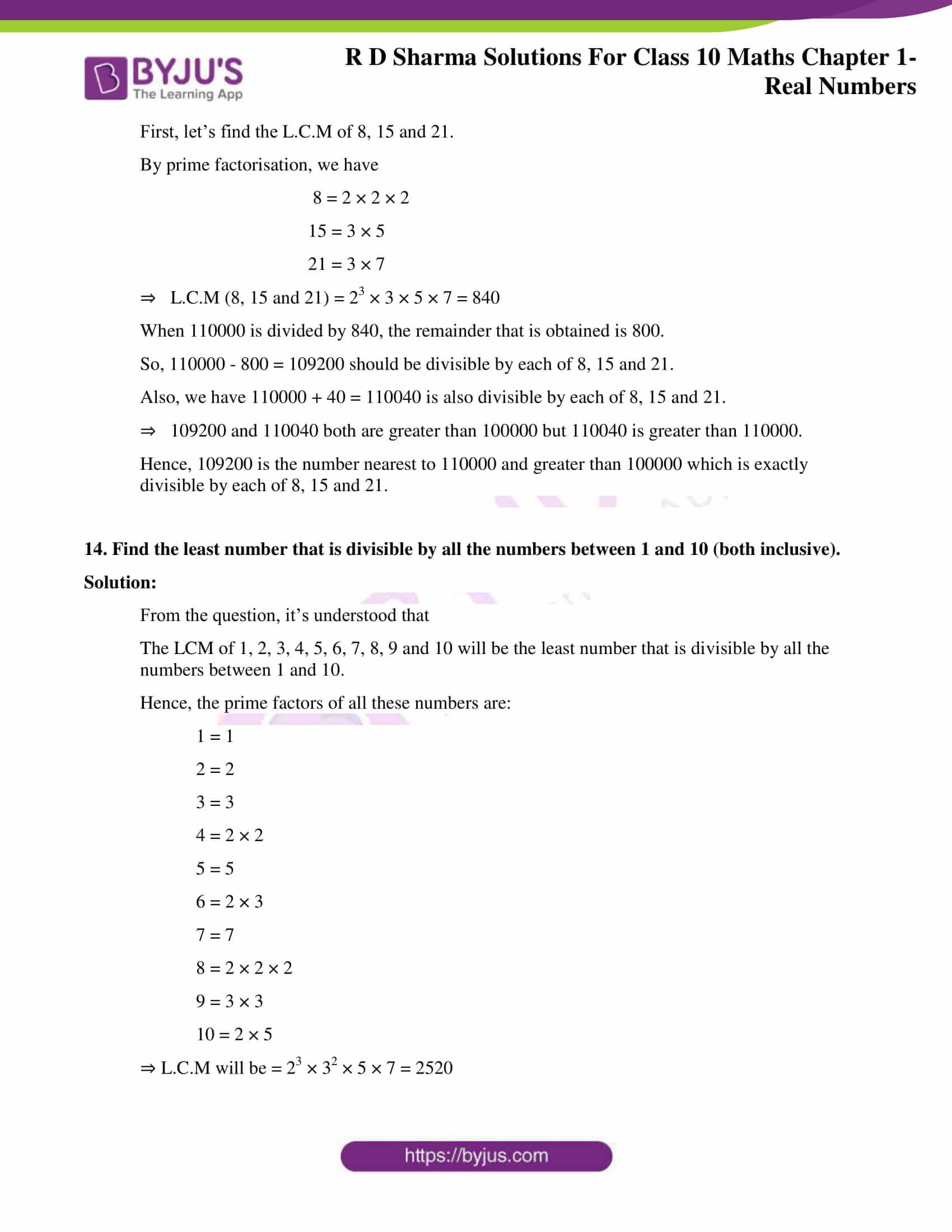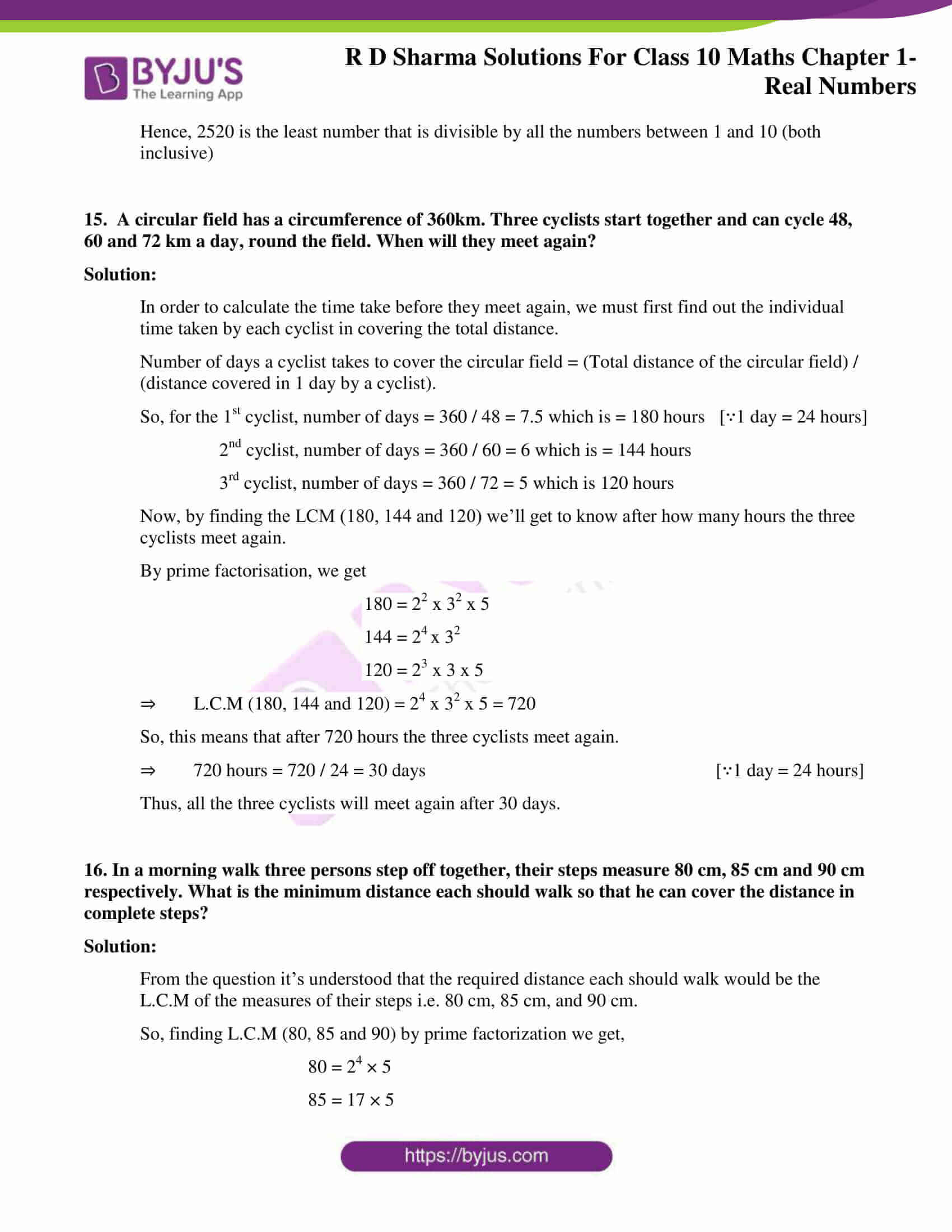### Access answers to Maths RD Sharma Solutions for Class 10 Chapter 1 Real Numbers Exercise 1.4

1. Find the LCM and HCF of the following pairs of integers and verify that LCM × HCF = Product of the integers:

(i) 26 and 91

Solution:

Given integers are: 26 and 91

First, find the prime factors of 26 and 91.

26 = 2 × 13

91 = 7 × 13

∴ L.C.M (26, 91) = 2 × 7 × 13 = 182

And,

H.C.F (26, 91) = 13

Verification:

L.C.M × H.C.F = 182 x 13= 2366

And, product of the integers = 26 x 91 = 2366

∴ L.C.M × H.C.F = product of the integers

Hence verified.

(ii) 510 and 92

Solution:

Given integers are: 510 and 92

First, find the prime factors of 510 and 92.

510 = 2 × 3 × 5 × 17

92 = 2 × 2 × 23

∴ L.C.M (510, 92) = 2 × 2 × 3 × 5 × 23 × 17 = 23460

And,

H.C.F (510, 92) = 2

Verification:

L.C.M × H.C.F = 23460 x 2 = 46920

And, product of the integers = 510 x 92 = 46920

∴ L.C.M × H.C.F = product of the integers

Hence verified.

(iii) 336 and 54

Solution:

Given integers are: 336 and 54

First, find the prime factors of 336 and 54.

336 = 2 × 2 × 2 × 2 × 3 × 7

54 = 2 × 3 × 3 x 3

∴ L.C.M (336, 54) = 2 × 2 × 2 × 2 × 3 × 3 × 3 × 7 = 3024

And,

H.C.F (336, 54) = 2 x 3 = 6

Verification:

L.C.M × H.C.F = 3024 x 6 = 18144

And, product of the integers = 336 x 54 = 18144

∴ L.C.M × H.C.F = product of the integers

Hence verified.

2. Find the LCM and HCF of the following integers by applying the prime factorization method:

(i) 12, 15 and 21

Solution:

First, find the prime factors of the given integers: 12, 15 and 21

For, 12 = 2 × 2 × 3

15 = 3 × 5

21 = 3 × 7

Now, L.C.M of 12, 15 and 21 = 2 × 2 × 3 × 5 × 7

∴ L.C.M (12, 15, 21) = 420

And, H.C.F (12, 15 and 21) = 3

(ii) 17, 23 and 29

Solution:

First, find the prime factors of the given integers: 17, 23 and 29

For, 17 = 1 × 17

23 = 1 × 23

29 = 1 × 29

Now, L.C.M of 17, 23 and 29 = 1 × 17 × 23 × 29

∴ L.C.M (17, 23, 29) = 11339

And, H.C.F (17, 23 and 29) = 1

(iii) 8, 9 and 25

Solution:

First, find the prime factors of the given integers: 8, 9 and 25

For, 8 = 2 × 2 x 2

9 = 3 × 3

25 = 5 × 5

Now, L.C.M of 8, 9 and 25 = 23 × 32 × 52

∴ L.C.M (8, 9, 25) = 1800

And, H.C.F (8, 9 and 25) = 1

(iv) 40, 36 and 126

Solution:

First, find the prime factors of the given integers: 40, 36 and 126

For, 40 = 2 x 2 x 2 × 5

36 = 2 x 2 x 3 x 3

126 = 2 × 3 × 3 × 7

Now, L.C.M of 40, 36 and 126 = 23 × 32 × 5 × 7

∴ L.C.M (40, 36, 126) = 2520

And, H.C.F (40, 36 and 126) = 2

(v) 84, 90 and 120

Solution:

First, find the prime factors of the given integers: 84, 90 and 120

For, 84 = 2 × 2 × 3 × 7

90 = 2 × 3 × 3 × 5

120 = 2 × 2 × 2 × 3 × 5

Now, L.C.M of 84, 90 and 120 = 23 × 32 × 5 × 7

∴ L.C.M (84, 90, 120) = 2520

And, H.C.F (84, 90 and 120) = 6

(vi) 24, 15 and 36

Solution:

First, find the prime factors of the given integers: 24, 15 and 36

For, 24 = 2 × 2 x 2 x 3

15 = 3 × 5

36 = 2 × 2 × 3 × 3

Now, LCM of 24, 15 and 36 = 2 × 2 × 2 × 3 × 3 × 5 = 23 x 32 x 5

∴ LCM (24, 15, 36) = 360

And, HCF (24, 15 and 36) = 3

3. Given that HCF (306, 657) = 9 , find LCM ( 306, 657 )

Solution:

Given two integers are: 306 and 657

We know that,

LCM × HCF = Product of the two integers

⇒ LCM = Product of the two integers / HCF

= (306 x 657) / 9 = 22338

4. Can two numbers have 16 as their HCF and 380 as their LCM? Give reason.

Solution:

On dividing 380 by 16 we get,

23 as the quotient and 12 as the remainder.

Now, since the LCM is not exactly divisible by the HCF its can be said that two numbers cannot have 16 as their HCF and 380 as their LCM.

5. The HCF of two numbers is 145 and their LCM is 2175. If one number is 725, find the other.

Solution:

The LCM and HCF of two numbers are 145 and 2175 respectively. (Given)

It is also given that, one of the numbers is 725

We know that,

LCM × HCF = first number × second number

2175 × 145  = 725 × second number

⇒ Second number = (2175 × 145)/ 725 = 435

∴ the other number is 435.

6. The HCF of two numbers is 16 and their product is 3072. Find their LCM.

Solution:

Given,

HCF of two numbers = 16

And, their product = 3072

We know that,

LCM × HCF = Product of the two numbers

LCM × 16 = 3072

⇒ LCM = 3072/ 16 = 192

∴ the LCM of the two numbers is 192.

7. The LCM and HCF of two numbers are 180 and 6 respectively. If one of the numbers is 30, find the other number.

Solution:

Given,

The LCM and HCF of two numbers are 180 and 6 respectively. (Given)

It is also given that, one of the numbers is 30.

We know that,

LCM × HCF = first number × second number

180 × 6 = 30 × second number

⇒ Second number = (180 × 6)/ 30 = 36

∴ the other number is 36.

8. Find the smallest number which when increased by 17 is exactly divisible by both 520 and 468.

Solution:

First let’s find the smallest number which is exactly divisible by both 520 and 468.

That is simply just the LCM of the two numbers.

By prime factorisation, we get

520 = 23 × 5 × 13

468 = 22 × 32 × 13

∴ LCM (520, 468) = 23 × 32 × 5 × 13 = 4680

Hence, 4680 is the smallest number which is exactly divisible by both 520 and 468 i.e. we will get a remainder of 0 in each case. But, we need to find the smallest number which when increased by 17 is exactly divided by 520 and 468.

So that is found by,

4680 – 17 = 4663

∴ 4663 should be the smallest number which when increased by 17 is exactly divisible by both 520 and 468.

9. Find the smallest number which leaves remainders 8 and 12 when divided by 28 and 32 respectively.

Solution:

First, let’s find the smallest number which is exactly divisible by both 28 and 32.

Which is simply just the LCM of the two numbers.

By prime factorisation, we get

28 = 2 × 2 × 7

32 = 25

∴ L.C.M (28, 32) = 25 × 7 = 224

Hence, 224 is the smallest number which is exactly divisible by 28 and 32 i.e. we will get a remainder of 0 in each case. But, we need the smallest number which leaves remainders 8 and 12 when divided by 28 and 32 respectively.

So that is found by,

224 – 8 – 12 = 204

∴ 204 should be the smallest number which leaves remainders 8 and 12 when divided by 28 and 32 respectively.

10. What is the smallest number that, when divided by 35, 56 and 91 leaves remainders of 7 in each case?

Solution:

First, let’s find the smallest number which is exactly divisible by all 35, 56 and 91.

Which is simply just the LCM of the three numbers.

By prime factorisation, we get

35 = 5 × 7

56 = 23 × 7

91 = 13 × 7

∴ L.C.M (35, 56 and 91) = 23 × 7 × 5 × 13 = 3640

Hence, 3640 is the smallest number that when divided by 35, 56 and 91 leaves the remainder of 7 in each case.

So that is found by,

3640 + 7 = 3647

∴ 3647 should be the smallest number that when divided by 35, 56 and 91 leaves the remainder of 7 in each case.

11. A rectangular courtyard is 18m 72cm long and 13m 20 cm broad. It is to be paved with square tiles of the same size. Find the least possible number of such tiles.

Solution:

Given,

Length of courtyard = 18 m 72 cm = 1800 cm + 72 cm = 1872 cm (∵1 m = 100 cm)

Breadth of courtyard = 13 m 20 cm = 1300 cm + 20 cm = 1320 cm

The size of the square tile needed to be paved on the rectangular yard is equal to the HCF of the length and breadth of the rectangular courtyard.

Now, finding the prime factors of 1872 and 1320, we have

1872 = 24 × 32 × 13

1320 = 23 × 3 × 5 × 11

⇒ HCF (1872 and 1320) = 23 × 3 = 24

∴ the length of side of the square tile should be 24 cm.

Thus, the number of tiles required = (area of the courtyard) / (area of a square tile)

We know that, area of the courtyard = Length × Breadth

= 1872 cm × 1320 cm

And, area of a square tile = (side)2 = (24cm)2

⇒ the number of tiles required = (1872 x 1320) / (24)2 = 4290

Thus, the least possible number of tiles required is 4290.

12. Find the greatest number of 6 digits exactly divisible by 24, 15 and 36.

Solution:

We know that, the greatest 6 digit number is 999999.

Let’s assume that 999999 is divisible by 24, 15 and 36 exactly.

Then, the LCM (24, 15 and 36) should also divide 999999 exactly.

Finding the prime factors of 24, 15, and 36, we get

24 = 2 × 2 × 2 × 3

15 = 3 × 5

36 = 2 × 2 × 3 × 3

⇒ L.C.M of 24, 15 and 36 = 360

Since, (999999)/ 360 = 2777 × 360 + 279

Here, the remainder is 279.

So, the greatest number which is divisible by all three should be = 999999 – 279 = 999720

∴ 999720 is the greatest 6 digit number which is exactly divisible by 24, 15 and 36.

13. Determine the number nearest to 110000 but greater 100000 which is exactly divisible by each of 8, 15 and 21.

Solution:

First, let’s find the L.C.M of 8, 15 and 21.

By prime factorisation, we have

8 = 2 × 2 × 2

15 = 3 × 5

21 = 3 × 7

⇒ L.C.M (8, 15 and 21) = 23 × 3 × 5 × 7 = 840

When 110000 is divided by 840, the remainder that is obtained is 800.

So, 110000 – 800 = 109200 should be divisible by each of 8, 15 and 21.

Also, we have 110000 + 40 = 110040 is also divisible by each of 8, 15 and 21.

⇒ 109200 and 110040 both are greater than 100000 but 110040 is greater than 110000.

Hence, 109200 is the number nearest to 110000 and greater than 100000 which is exactly divisible by each of 8, 15 and 21.

14. Find the least number that is divisible by all the numbers between 1 and 10 (both inclusive).

Solution:

From the question, it’s understood that

The LCM of 1, 2, 3, 4, 5, 6, 7, 8, 9 and 10 will be the least number that is divisible by all the numbers between 1 and 10.

Hence, the prime factors of all these numbers are:

1 = 1

2 = 2

3 = 3

4 = 2 × 2

5 = 5

6 = 2 × 3

7 = 7

8 = 2 × 2 × 2

9 = 3 × 3

10 = 2 × 5

⇒ L.C.M will be = 23 × 32 × 5 × 7 = 2520

Hence, 2520 is the least number that is divisible by all the numbers between 1 and 10 (both inclusive)

15.  A circular field has a circumference of 360km. Three cyclists start together and can cycle 48, 60 and 72 km a day, round the field. When will they meet again?

Solution:

In order to calculate the time take before they meet again, we must first find out the individual time taken by each cyclist in covering the total distance.

Number of days a cyclist takes to cover the circular field = (Total distance of the circular field) / (distance covered in 1 day by a cyclist).

So, for the 1st cyclist, number of days = 360 / 48 = 7.5 which is = 180 hours [∵1 day = 24 hours]

2nd cyclist, number of days = 360 / 60 = 6 which is = 144 hours

3rd cyclist, number of days = 360 / 72 = 5 which is 120 hours

Now, by finding the LCM (180, 144 and 120) we’ll get to know after how many hours the three cyclists meet again.

By prime factorisation, we get

180 = 22 x 32 x 5

144 = 24 x 32

120 = 23 x 3 x 5

⇒ L.C.M (180, 144 and 120) = 24 x 32 x 5 = 720

So, this means that after 720 hours the three cyclists meet again.

⇒ 720 hours = 720 / 24 = 30 days [∵1 day = 24 hours]

Thus, all the three cyclists will meet again after 30 days.

16. In a morning walk three persons step off together, their steps measure 80 cm, 85 cm and 90 cm respectively. What is the minimum distance each should walk so that he can cover the distance in complete steps?

Solution:

From the question it’s understood that the required distance each should walk would be the L.C.M of the measures of their steps i.e. 80 cm, 85 cm, and 90 cm.

So, finding L.C.M (80, 85 and 90) by prime factorization we get,

80 = 24 × 5

85 = 17 × 5

90 = 2 × 3 × 3 × 5

⇒ L.C.M (80, 85 and 90) = 24 × 32 × 5 × 17 = 12240 cm = 122m 40cm [∵1 m = 100cm]

Hence, 122 m 40 cm is the minimum distance that each should walk so that all can cover the same distance in complete steps.